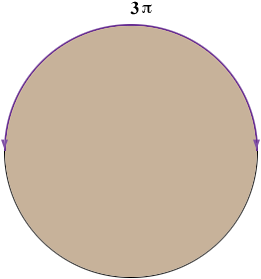SEARCH HOMEMath Central Quandaries & QueriesQuestion from grier, a student: please, what is the area of a semicircle with arc length 3pi?Hi Grier,

If the arc length of half a circle is 3 π, what is the arc length (circumference) of the entire circle?The circumference of a circle is 2 × π × (the radius) so what is the radius of the circle? What is the formula for the area of a circle? What is the area of this circle?

PennyMath Central is supported by the University of Regina and The Pacific Institute for the Mathematical Sciences.